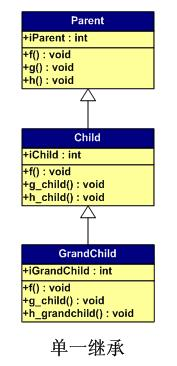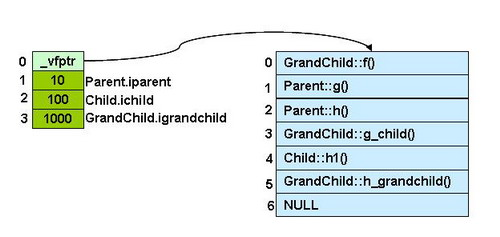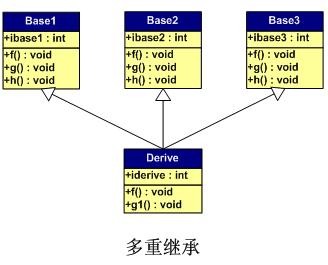C++ 对象的内存布局(上)

# 前言

0712月，我写了一篇《C++虚函数表解析》的文章，引起了大家的兴趣。有很多朋友对我的文章留了言，有鼓励我的，有批评我的，还有很多问问题的。我在这里一并对大家的留言表示感谢。这也是我为什么再写一篇续言的原因。因为，在上一篇文章中，我用了的示例都是非常简单的，主要是为了说明一些机理上的问题，也是为了图一些表达上方便和简单。不想，这篇文章成为了打开C++对象模型内存布局的一个引子，引发了大家对C++对象的更深层次的讨论。当然，我之前的文章还有很多方面没有涉及，从我个人感觉下来，在谈论虚函数表里，至少有以下这些内容没有涉及：

1）有成员变量的情况。

2）有重复继承的情况。

3）有虚拟继承的情况。

4）有钻石型虚拟继承的情况。

# 对象的影响因素

1）成员变量

2）虚函数（产生虚函数表）

3）单一继承（只继承于一个类）

4）多重继承（继承多个类）

5）重复继承（继承的多个父类中其父类有相同的超类）

6）虚拟继承（使用virtual方式继承，为了保证继承后父类的内存布局只会存在一份）

1）单一的一般继承（带成员变量、虚函数、虚函数覆盖）

2）单一的虚拟继承（带成员变量、虚函数、虚函数覆盖）

3）多重继承（带成员变量、虚函数、虚函数覆盖）

4）重复多重继承（带成员变量、虚函数、虚函数覆盖）

5）钻石型的虚拟多重继承（带成员变量、虚函数、虚函数覆盖）

# 知识复习

typedef void(*Fun)(void);

Base b;

Fun pFun = NULL;

cout << "虚函数表地址：" << (int*)(&b) << endl;

cout << "虚函数表第一个函数地址：" << (int*)*(int*)(&b) << endl;

// Invoke the first virtual function

pFun = (Fun)*((int*)*(int*)(&b));

pFun();

1）Windows XP VC++ 2003

2）Cygwin G++ 3.4.4

# 单一的一般继承class Parent {

public:

int iparent;

Parent ():iparent (10) {}

virtual void f() { cout << " Parent::f()" << endl; }

virtual void g() { cout << " Parent::g()" << endl; }

virtual void h() { cout << " Parent::h()" << endl; }

};

class Child : public Parent {

public:

int ichild;

Child():ichild(100) {}

virtual void f() { cout << "Child::f()" << endl; }

virtual void g_child() { cout << "Child::g_child()" << endl; }

virtual void h_child() { cout << "Child::h_child()" << endl; }

};

class GrandChild : public Child{

public:

int igrandchild;

GrandChild():igrandchild(1000) {}

virtual void f() { cout << "GrandChild::f()" << endl; }

virtual void g_child() { cout << "GrandChild::g_child()" << endl; }

virtual void h_grandchild() { cout << "GrandChild::h_grandchild()" << endl; }

};

typedef void(*Fun)(void);

GrandChild gc;

int** pVtab = (int**)&gc;

cout << " GrandChild::_vptr->" << endl;

for (int i=0; (Fun)pVtab[i]!=NULL; i++){

pFun = (Fun)pVtab[i];

cout << "    ["<<i<<"] ";

pFun();

}

cout << " Parent.iparent = " << (int)pVtab << endl;

cout << " Child.ichild = " << (int)pVtab << endl;

cout << " GrandChild.igrandchild = " << (int)pVtab << endl;

  GrandChild::_vptr->      GrandChild::f()      Parent::g()      Parent::h()      GrandChild::g_child()      Child::h1()      GrandChild::h_grandchild()  Parent.iparent = 10  Child.ichild = 100  GrandChild.igrandchild = 10001）虚函数表在最前面的位置。

2）成员变量根据其继承和声明顺序依次放在后面。

3）在单一的继承中，被overwrite的虚函数在虚函数表中得到了更新。

# 多重继承class Base1 {

public:

int ibase1;

Base1():ibase1(10) {}

virtual void f() { cout << "Base1::f()" << endl; }

virtual void g() { cout << "Base1::g()" << endl; }

virtual void h() { cout << "Base1::h()" << endl; }

};

class Base2 {

public:

int ibase2;

Base2():ibase2(20) {}

virtual void f() { cout << "Base2::f()" << endl; }

virtual void g() { cout << "Base2::g()" << endl; }

virtual void h() { cout << "Base2::h()" << endl; }

};

class Base3 {

public:

int ibase3;

Base3():ibase3(30) {}

virtual void f() { cout << "Base3::f()" << endl; }

virtual void g() { cout << "Base3::g()" << endl; }

virtual void h() { cout << "Base3::h()" << endl; }

};

class Derive : public Base1, public Base2, public Base3 {

public:

int iderive;

Derive():iderive(100) {}

virtual void f() { cout << "Derive::f()" << endl; }

virtual void g1() { cout << "Derive::g1()" << endl; }

};

typedef void(*Fun)(void);

Derive d;

int** pVtab = (int**)&d;

cout << " Base1::_vptr->" << endl;

pFun = (Fun)pVtab;

cout << "      ";

pFun();

pFun = (Fun)pVtab;

cout << "      ";pFun();

pFun = (Fun)pVtab;

cout << "      ";pFun();

pFun = (Fun)pVtab;

cout << "      "; pFun();

pFun = (Fun)pVtab;

cout << "      "; cout<<pFun<<endl;

cout << " Base1.ibase1 = " << (int)pVtab << endl;

int s = sizeof(Base1)/4;

cout << "[" << s << "] Base2::_vptr->"<<endl;

pFun = (Fun)pVtab[s];

cout << "      "; pFun();

Fun = (Fun)pVtab[s];

cout << "      "; pFun();

pFun = (Fun)pVtab[s];

cout << "      "; pFun();

pFun = (Fun)pVtab[s];

out << "      ";

cout<<pFun<<endl;

cout << "["<< s+1 <<"] Base2.ibase2 = " << (int)pVtab[s+1] << endl;

s = s + sizeof(Base2)/4;

cout << "[" << s << "] Base3::_vptr->"<<endl;

pFun = (Fun)pVtab[s];

cout << "      "; pFun();

pFun = (Fun)pVtab[s];

cout << "      "; pFun();

pFun = (Fun)pVtab[s];

cout << "      "; pFun();

pFun = (Fun)pVtab[s];

cout << "      ";

cout<<pFun<<endl;

s++;

cout << "["<< s <<"] Base3.ibase3 = " << (int)pVtab[s] << endl;

s++;

cout << "["<< s <<"] Derive.iderive = " << (int)pVtab[s] << endl;

  Base1::_vptr->       Derive::f()       Base1::g()       Base1::h()       Driver::g1()       00000000      ç 注意：在GCC下，这里是1  Base1.ibase1 = 10  Base2::_vptr->       Derive::f()       Base2::g()       Base2::h()       00000000      ç 注意：在GCC下，这里是1  Base2.ibase2 = 20  Base3::_vptr->       Derive::f()       Base3::g()       Base3::h()       00000000  Base3.ibase3 = 30  Derive.iderive = 1001）  每个父类都有自己的虚表。

2）  子类的成员函数被放到了第一个父类的表中。

3）  内存布局中，其父类布局依次按声明顺序排列。

4）  每个父类的虚表中的f()函数都被overwrite成了子类的f()。这样做就是为了解决不同的父类类型的指针指向同一个子类实例，而能够调用到实际的函数。

(转载时请注明作者和出处。未经许可，请勿用于商业用途)

07-24
04-27299401-147647
10-156万+
10-172154
04-11604
08-041565
10-25401
08-143308